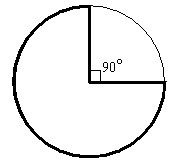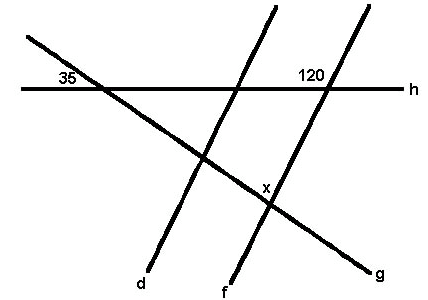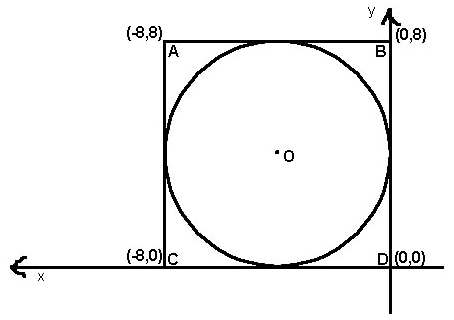+91-22-62437100 / 01 | help@reachivy.com

# SAT Math Quiz 2

Posted on Oct 16, 2017

To begin click Next

Question 1:

Which of the following sets of three numbers could be the side lengths, in yards, of a right triangle containing a 45° angle?

Question 2:There is to be a walkway built around a circular pond. The walkway will include a curved section extending most of the way around the pond and two straight sections, each measuring 15 feet, extending from the edge that meet in the center at a 90 degree angle to each other, as shown in the figure. Which of the following is closest to the total length of the walkway, in feet?
Question 3:

Pollcorp is making a circle graph to illustrate the results of their recent poll of voters about the upcoming mayoral election. 25% of voters support Kendra Willard; 20% support Steve Jacobson; 15% support Amber Tarkington; and 10% support Harry Fink. The remaining voters either expressed no preference or supported other candidates. These voters will be grouped together into the category “Other Response.” What will be the degree measure of the Other Response sector?

Question 4:

At summer camp, Ryan is making a tablecloth for a circular table that is 3 feet in diameter. The finished tablecloth must hang down 4 inches over the edge of the table all the way around. Ryan will also need to fold under and sew down 2 inches of material all around the edge of the tablecloth. Ryan plans to use a single piece of square fabric that is 75 inches long. What is the longest width of uncut fabric, in inches, that could be left over?

Question 5:In the figure to the left, lines d and f are parallel and the angle measures are as given. What is the value of x?

Question 6:The vertices of square ABCD have coordinates (0,0), (-8,0), (-8,8), and (0,8) in the standard (x,y) coordinate plane to the left, and circle O is inscribed in square ABCD. Which of the following equations represents circle O?

Question 7:

A and B are reciprocals (when multiplied together their product is 1). If A < -1, then B must be which of the following?

Question 8:

How many of the numbers between 20 and 40 are prime numbers?

Question 9:

If AB = BC and DB = BE, which of the following is not necessarily true?

Question 10:

The two parallel sides of an isoceles trapazoid are 8 inches apart. If one of the parallel sides is 12 inches, what is the length of the other side, x?

You have answered all the questions in the quiz. Press Submit to view your score